Courses

# Test: Epoxides

## 22 Questions MCQ Test Chemistry Class 12 | Test: Epoxides

Description
This mock test of Test: Epoxides for Class 12 helps you for every Class 12 entrance exam. This contains 22 Multiple Choice Questions for Class 12 Test: Epoxides (mcq) to study with solutions a complete question bank. The solved questions answers in this Test: Epoxides quiz give you a good mix of easy questions and tough questions. Class 12 students definitely take this Test: Epoxides exercise for a better result in the exam. You can find other Test: Epoxides extra questions, long questions & short questions for Class 12 on EduRev as well by searching above.
QUESTION: 1

### Only One Option Correct Type Direction (Q. Nos. 1-8) This section contains 8 multiple choice questions. Each question has four choices (a), (b), (c) and (d), out of which ONLY ONE is correct. Q. The most appropriate reagents that can bring about the following transformation is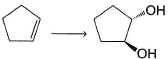Solution:

Epoxide upon hydrolysis gives anti, vicinal diois.

QUESTION: 2

### Which major organic product is formed in the following reaction ?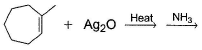Solution:

Epoxide undergo nucleophilic attack of NH3 at less substituted α-carbon giving anti hydroxy amine.

QUESTION: 3

### The major organic product formed in the reaction given below is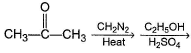Solution: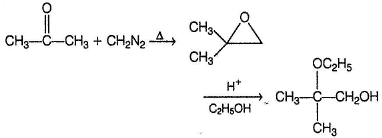QUESTION: 4

In the following hydrolysis reactio, the major final product is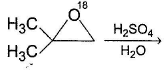Solution: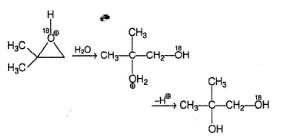On protonated epoxide, nucleophilic attack occur at α-carbon Where more stable carbocation could be formed.

QUESTION: 5

In the reaction given below,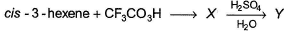The correct deduction concerning X and Y is

Solution: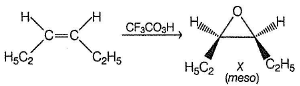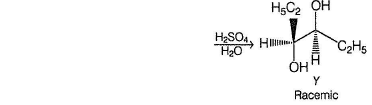QUESTION: 6

An alkene on treatment with meta chloro perbenzoic acid undergoes epoxidation reaction to give oxirane. What is the correct order of increasing reactivity of the following in the above mentioned epoxidation reaction?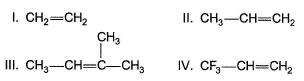Solution:

Epoxidation is an example of electrophilic ad dition at olefin ic bonds. Therefore, greater the electron density at olefinic bond, greater its reactivity. Electron donating groups increases reactivity while electron withdrawing group s like — CF3 decreases reactivity.

QUESTION: 7

Which of the following compound gives more than one oxirane upon epoxidation with Ag2O/heat?

Solution:

It gives racemic mixture of oxiranes.

QUESTION: 8

The reaction given beiow that gives oxirane as the major organic product is

Solution: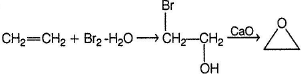*Multiple options can be correct
QUESTION: 9

One or More than One Options Correct Type

Direction (Q, Nos. 9-14)This section contains 6 multiple type questions. Each question has 4 choices (a), (b), (c) and (d), out of which ONE or MORE THAN ONE are correct.

Consider the following reaction sequence,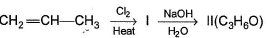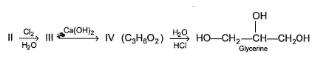Q.

The intermediate products (I - IV) in the above reaction is/are

Solution: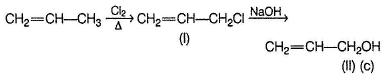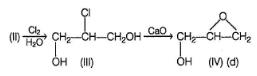*Multiple options can be correct
QUESTION: 10

The reagent that can bring about the following transformation in good yield is/are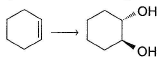Solution:

(c) Epoxide on hydrolysis gives an ti diol.
(d) Halohydrin is formed in first step which gives epoxide with CaO. Epoxide is then hydrolysed in H2SO4 giving anti diol.

*Multiple options can be correct
QUESTION: 11

In the reaction below,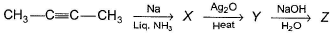The correct statement concerning the above reaction is/are

Solution: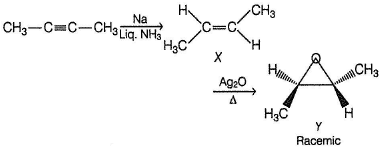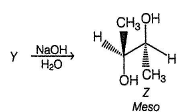*Multiple options can be correct
QUESTION: 12

Consider the reaction given below,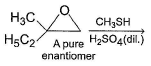Solution:

The m ajor product would be the only enantiom er form ed from a given enantiomer of starting compound.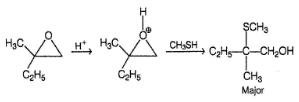*Multiple options can be correct
QUESTION: 13

Consider the following reaction sequence,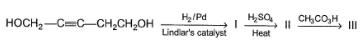I, II and III could be

Solution: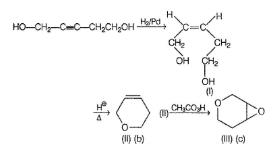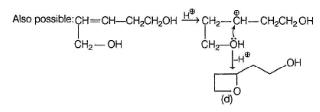*Multiple options can be correct
QUESTION: 14

Consider the following reaction sequence,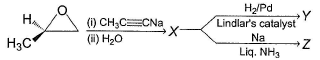The correct statem ent regarding X, Y and Z is/are

Solution: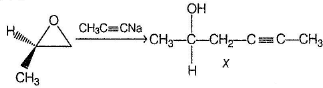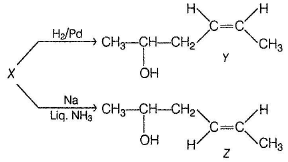Since, a pure enantiomer of oxirane is taken, a pure enantiomer of X would be produced. Y and Z are diastereomers as they are not enantiomers but stereoisomers.

QUESTION: 15

Comprehension Type

Direction (Q. Nos. 15-17) This section contains a passage describing theory, experiments, data, etc. Two questions related to the paragraph have been given. Each question has only one correct answer out of the given 4 options (a), (b), (c) and (d).

Passage

Oxirane is a reactive ether, undergo nucleophilic attack at a-carbon in the presence of both acidic and basic medium. The generalised mechanism in the two medium are :

I. Acidic medium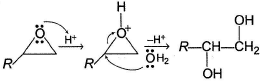II. Basic medium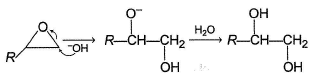As shown above, in acidic medium, nucleophilic attack occur at more substituted α-carbon while in basic medium, nucleophilic attack occur preferably at less substituted α-carbon.

Q.

In the reaction given below, th e final major organic product Y is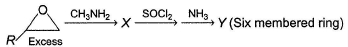Solution: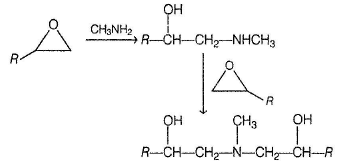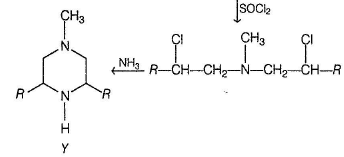QUESTION: 16

Oxirane is a reactive ether, undergo nucleophilic attack at a-carbon in the presence of both acidic and basic medium. The generalised mechanism in the two medium are :

I. Acidic mediumII. Basic mediumAs shown above, in acidic medium, nucleophilic attack occur at more substituted α-carbon while in basic medium, nucleophilic attack occur preferably at less substituted α-carbon.

Q.

If X is heated with concentrated H2SO4 a cyclic compound is formed which is

Solution: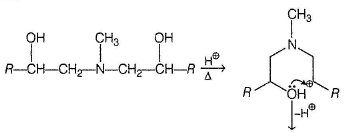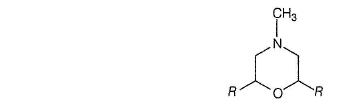QUESTION: 17

Oxirane is a reactive ether, undergo nucleophilic attack at a-carbon in the presence of both acidic and basic medium. The generalised mechanism in the two medium are :

I. Acidic mediumII. Basic mediumAs shown above, in acidic medium, nucleophilic attack occur at more substituted α-carbon while in basic medium, nucleophilic attack occur preferably at less substituted α-carbon.

Q.

What would be formed if 2-phenyloxirane is treated with CH3MgBr followed by acid hydrolysis?

Solution: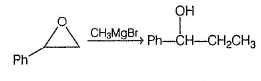*Answer can only contain numeric values
QUESTION: 18

One Integer Value Correct Type

Direction (Q. Nos. 18-22) This section contains 5 questions. When worked out will result in an integer from 0 to 9 (both inclusive).

Q.

How many different ether isomers are possible for C3H6O ?

Solution: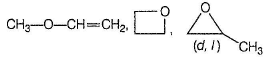*Answer can only contain numeric values
QUESTION: 19

Consider the reaction given below,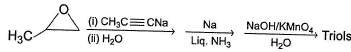Q.

How many different triols isomers are formed?

Solution: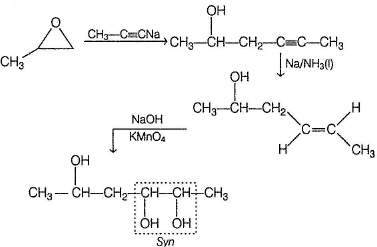*Answer can only contain numeric values
QUESTION: 20

If propene undergoes oxidation to methyl oxirane, what is the net change in oxidation number of carbon atoms?

Solution: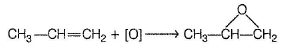Oxidation number of both carbons of oxirane ring increases by one unit each.

*Answer can only contain numeric values
QUESTION: 21

In the following reaction,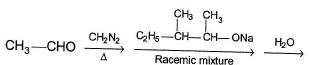Q.

How many different stereoisomers of product formed ?

Solution: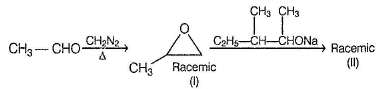Each enantiomer of (I) will react with racemic mixture of (II) forming a pair of diastereomers.

*Answer can only contain numeric values
QUESTION: 22

How many different arene oxide would be formed on mono epoxidation of phenanthrene?

Solution: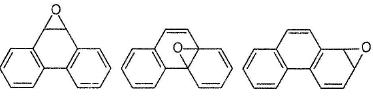• Test